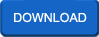## Engineering Thermodynamics

 Thermodynamic Property RelationsSome thermodynamic properties can be measured directly, but many others cannot. Therefore, it is necessary to develop some relations between these two groups so that the properties that cannot be measured directly can be evaluated.
Rate this Book:
 Solution ThermodynamicsReal system usually contains a mixture of fluid. Develop the theoretical foundation for applications of thermodynamics to gas mixtures and liquid solutions
Rate this Book:
 Irreversible Isothermal ExpansionWork in an Isothermal Expansion: Approaching Reversibility,etc..,
Rate this Book:
 PsychrometryThe Trane Company believes that it is incumbent on manufacturers to serve the industry by regularly disseminating information gathered through laboratory research, testing programs, and field experience.
Rate this Book:
 Psychrometric FundamentalsUnderstand the significance of psychrometrics to “air conditioning”,Understand common psychrometric terms including: dry bulb and wet bulb temperature, relative humidity, specific humidity, dew point,enthalpy, flow rate, & “standard air”, Identify all lines and units of measure on the psychrometric chart
Rate this Book:
 Maxwell RelationsFirst, start with a known equation of state such as that of internal energy
Rate this Book:
 Joule-Thomson CoefficientThe objective of this experiment is to quantitatively measure the non-ideality of gases using the Joule-Thomson coefficient and relating it to the coefficients of equations for non-ideality and the Lennard-Jones potential.
Rate this Book:
 First Law Of ThermodynamicsJames Joule showed that mechanical energy could be converted to heat and arrived at the conclusion that heat was another form of energy
Rate this Book:
 EntropyIntroduce the thermodynamic property entropy (S) using the Clausius inequality Recognize the fact that the entropy is always increasing for an isolated system (or a system plus its surroundings) based on the increase of entropy principle
Rate this Book:
 Energy Conservation In Steady FlowThe sketch above shows a piece of equipment such as a boiler, engine, pump, etc. through which fluid steadily flows. The fluid enters the equipment with velocity V1 at an inlet 1 with area A1 and leaves with velocity V2 by an exhaust at 2 with area A2 .
Rate this Book:
 States of Matter; Liquids and SolidsPhase transitions,Phase Diagrams,Liquid State,Properties of Liquids; Surface tension and viscosity Intermolecular forces; explaining liquid properties etc..,
Rate this Book:
 Concepts Of ThermodynamicsA system consists of two subsystems with the values U1, V1, P1 and U2, V2, P2, where U is the internal energy. U and V are both extensive quantities. The law of additivity applies to them and we have for the complete system U = U1 + U2; V = V1 + V2.
Rate this Book:
 Basic Concepts of Thermodynamicsthe system is the part of the world in which we have a special interest. A system has definite boundaries the surroundings is everything outside the boundaries
Rate this Book:
 The Second and Third Laws of ThermodynamicsDefinition of the Second Law,Determining DS,Definition of the Third Law
Rate this Book: